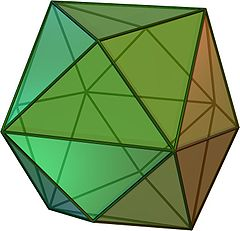Find a planar graph all of whose faces (including the outer face) are all quadrilaterals. Possible choices include the square, the cube, $$K_{2,n}$$, etc. (All such graphs are bipartite, a fact that will be useful below.) Turn it into a maximal planar graph by adding a new vertex inside each face, connected to all the vertices of the face. For instance, doing this to a cube produces a tetrakis cube, whose graph I've written about previously here. So for short, let's call the result of this construction a "tetrakis graph".It turns out that every tetrakis graph is a perfect graph, a graph for which the clique number of each induced subgraph equals its chromatic number. If the induced subgraph contains a triangle, or is independent, this is obvious, so the only interesting cases are the triangle-free induced subgraphs. They have clique number two so we need to check that they have chromatic number two. But the underlying quadrilateral-faced graph is always 2-colorable, and if there are no triangles then each of the added vertices that remains in the subgraph can only have neighbors of one color in this 2-coloring.

These aren't the only perfect maximal planar graphs: the tetrahedron $$K_4$$ is also perfect, and gluing two perfect maximal planar graphs together on a triangular face (which becomes a separating triangle in the glued graph) creates others. Gluing a collection of tetrahedra in this way produces an Apollonian network, and we can also glue together mixtures of tetrahedra and tetrakis graphs. But aside from the tetrahedron, are the tetrakis graphs the only building blocks needed to create the rest by gluing? That is, are they the only 4-connected perfect maximal planar graphs?

tomster0:
2013-11-27T03:51:55Z
Well, using your terminology, tetrakis graphs are the only maximal planar graphs whose vertices are properly 3-colorable. (There's a nice "3-color theorem" for planar graphs that implies this.) I think this answers your question in the affirmative.
11011110:
2013-11-27T04:19:07Z

I don't think it's true that they're the only 3-colorable maximal planar graphs. If you add a vertex into every face of a bipartite planar graph (regardless of whether its faces are all quadrilaterals) then you still get a 3-colorable graph. For instance, the truncated octahedron is bipartite (with square and regular hexagon faces) and if you add a vertex into each of its faces the result is 3-colorable but not perfect.

In the meantime I found another way to glue two perfect planar graphs together to get another perfect planar graph: cut off a degree-four vertex from each of them and glue the resulting quadrilaterals together. If the graphs are perfect, then in each of them every induced path between two opposite vertices of the gluing quadrilateral has the same parity, so you can't get any new odd holes when you glue them (and perfect planar graphs don't have odd antiholes because they're all nonplanar). Using this kind of gluing you can get some other graphs that are not themselves tetrakis.

So now the question is: is a non-tetrahedral perfect maximal planar graph without any separating triangles and without any two-colored separating quadrilaterals (in the unique 3-coloring of the graph) necessarily tetrakis?

tomster0:
2013-11-27T04:39:37Z